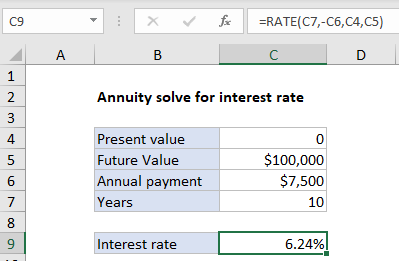## Excel Office

Excel How Tos, Tutorials, Tips & Tricks, Shortcuts

# How to calculate annuity for interest rate in excel

An annuity is a series of equal cash flows, spaced equally in time.  To solve for an annuity interest rate, you can use the RATE function.

Case study:  The goal in this example is to have \$100,000 at the end of 10 years, with an annual payment of \$7,500 made at the end of each year. What interest rate is required?

Worked Example:   Calculate interest rate for loan in Excel

## Formula

`=RATE(nper,pmt,pv,fv)`## Explanation

In the example shown C9 contains this formula:

`=RATE(C7,-C6,C4,C5)`

### Explanation

To solve for the interest rate, the RATE function is configured like this:

Worked Example:   PMT, RATE, NPER, PV and FV Financial Functions in Excel

nper – from cell C7, 10.
pmt – from cell C6, 7500 (negative sign)
pv – from cell C4, 0.
fv – from cell C5, 100000

Worked Example:   Calculate interest rate for loan in Excel

With this information, the RATE function returns 0.0624. Note payment is negative because it represents a cash outflow.